• 没关系，接下来我将以通俗的语言来详细解释梯度下降算法公式的数学推导过程。 下山问题 假设我们位于黄山的某个山腰处，山势连绵不绝，不知道怎么下山。于是决定走一步算一步，也就是每次沿着当前位...
原作者: 红色石头|来自: AI有道
梯度下降算法的公式非常简单，”沿着梯度的反方向（坡度最陡）“是我们日常经验得到的，其本质的原因到底是什么呢？为什么局部下降最快的方向就是梯度的负方向呢？也许很多朋友还不太清楚。没关系，接下来我将以通俗的语言来详细解释梯度下降算法公式的数学推导过程。
下山问题 假设我们位于黄山的某个山腰处，山势连绵不绝，不知道怎么下山。于是决定走一步算一步，也就是每次沿着当前位置最陡峭最易下山的方向前进一小步，然后继续沿下一个位置最陡方向前进一小步。这样一步一步走下去，一直走到觉得我们已经到了山脚。这里的下山最陡的方向就是梯度的负方向。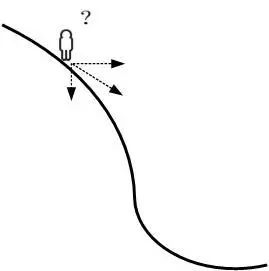首先理解什么是梯度？通俗来说，梯度就是表示某一函数在该点处的方向导数沿着该方向取得较大值，即函数在当前位置的导数。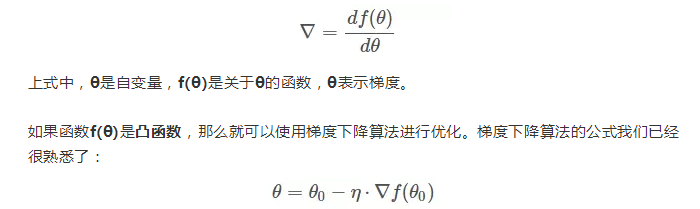其中，θo是自变量参数，即下山位置坐标，η是学习因子，即下山每次前进的一小步（步进长度），θ是更新后的θo，即下山移动一小步之后的位置。
一阶泰勒展开式 这里需要一点数学基础，对泰勒展开式有些了解。简单地来说，一阶泰勒展开式利用的就是函数的局部线性近似这个概念。我们以一阶泰勒展开式为例：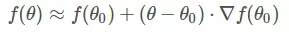不懂上面的公式？没有关系。我用下面这张图来解释。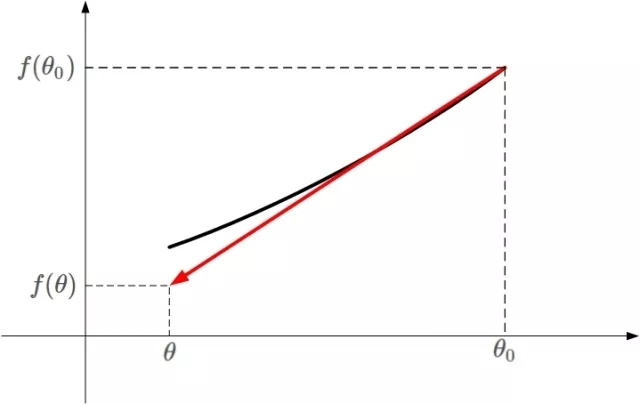凸函数f(θ)的某一小段[θo,θ]由上图黑色曲线表示，可以利用线性近似的思想求出f(θ)的值，如上图红色直线。该直线的斜率等于f(θ)在θo处的导数。则根据直线方程，很容易得到f(θ)的近似表达式为：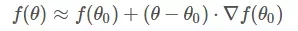这就是一阶泰勒展开式的推导过程，主要利用的数学思想就是曲线函数的线性拟合近似。
梯度下降数学原理 知道了一阶泰勒展开式之后，接下来就是重点了！我们来看一下梯度下降算法是如何推导的。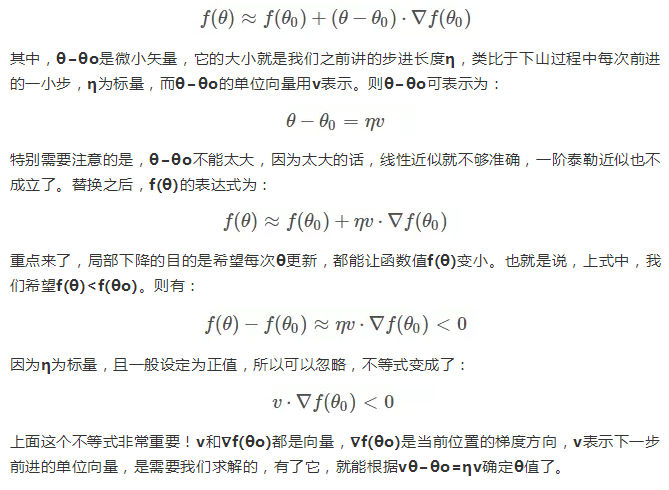想要两个向量的乘积小于零，我们先来看一下两个向量乘积包含哪几种情况：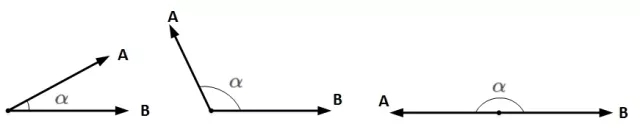A和B均为向量，α为两个向量之间的夹角。A和B的乘积为：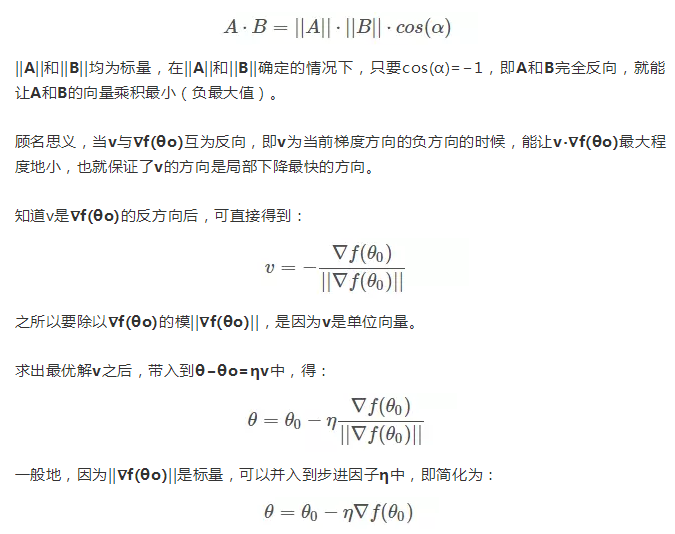总结 我们通过一阶泰勒展开式，利用线性近似和向量相乘最小化的思想搞懂了梯度下降算法的数学原理。
展开全文机器学习
• 梯度下降算法推导（机器学习系列-1）  在网上能够搜到很多关于梯度下降算法的文章，但找了几篇发现推导都不能很好的理解（也可能是愚生数学功底差），本文将着重从数学角度讲述一下梯度下降算法的数学推导。梯度...
  在网上能够搜到很多关于梯度下降算法的文章，但找了几篇发现推导都不能很好的理解（也可能是愚生数学功底差），本文将着重从数学角度讲述一下梯度下降算法的数学推导。

梯度下降算法理论
梯度下降算法源自于线性回归模型的cost function 最小值计算，在线性回归中，我们通过一个拟合函数：

h(θ)=θ0+θ1∗x1

h(\theta)=\theta_0+\theta_1*x_1，然后计算cost function：

J(θ)=12m∑i=0m(hθ(x)−y)2

J(\theta)=\frac{1}{2m}\sum_{i=0}^m(h_\theta(x)-y)^2
很明显这是计算在某一个

θ
$\theta$向量取值的时候，所得拟合函数在每组数据

x
$x$上的计算值与其实际值y$y$的差值，为了更好的展现这种误差，我们用平方和均值来表示，为了后面的计算方便还将其乘以

12
$\frac{1}{2}$。那么，后面的问题就是，当我们能够求得一组

θ
$\theta$值使得

J(θ)
$J(\theta)$得到最小值的时候，我们就认为得到了最佳拟合参数–

θ
$\theta$向量。因此，线性拟合模型的问题，最后就归结到了cost function的最小值计算了。那么这里要介绍的方法就是
梯度下降方法。我们通过梯度下降的方法来寻找

J(θ)
$J(\theta)$的最小值。
看过Andrew Ng视频的人肯定知道，梯度下降算法的原理，就是通过计算

J(θ)
$J(\theta)$的导数，通过寻找导数最小值的方式，来决定

J(θ)
$J(\theta)$的下降方向，在不断的迭代之后，即可找到

J(θ)
$J(\theta)$的最小值。以下就是

J(θ)
$J(\theta)$的求导计算：

J(θ)′=(12m∑i=0m(hθ(x)−y)2)′=12m(∑i=0m(hθ(x)−y)2)′=12m2(hθ(x)−y)∑i=0m(hθ(x)−y)′=1m(hθ(x)−y)∑i=0m(hθ)′=1m(hθ(x)−y)∑i=0m(θ0+θ1x1+θ2x2+...+θn)′

J(\theta)\prime=(\frac{1}{2m}\sum_{i=0}^m(h_\theta(x)-y)^2)\prime\\=\frac{1}{2m}(\sum_{i=0}^m(h_\theta(x)-y)^2)\prime\\=\frac{1}{2m}2(h_\theta(x)-y)\sum_{i=0}^m(h_\theta(x)-y)\prime\\=\frac{1}{m}(h_\theta(x)-y)\sum_{i=0}^m(h_\theta)\prime\\=\frac{1}{m}(h_\theta(x)-y)\sum_{i=0}^m(\theta_0+\theta_1x_1+\theta_2x_2+...+\theta_n)\prime
所以：

J(θj)′=1m(hθ(x)−y)∑i=0m(θ0+θ1x1+θ2x2+...+θn)′=1m(hθ(x)−y)xj

J(\theta_j)\prime=\frac{1}{m}(h_\theta(x)-y)\sum_{i=0}^m(\theta_0+\theta_1x_1+\theta_2x_2+...+\theta_n)\prime\\=\frac{1}{m}(h_\theta(x)-y)x_j

在梯度下降算法中，我们需要不断收敛各个

θj
$\theta_j$，寻找

J(θ)
$J(\theta)$的最小值。

θj
$\theta_j$在其导数方向上减少（下降），即可使得

J(θ)
$J(\theta)$达到最小值，最后当

J(θ)
$J(\theta)$收敛时，则停止

θj
$\theta_j$的计算。具体如下：

θ
$\theta$取随机值（初始值）    repeat:      计算

h(θ)
$h(\theta)$，将第一个样本数据y代入，更新

θj
$\theta_j$ -=

(h(θ)−y)θj
$(h(\theta)-y)\theta_j$，更新每个

θj
$\theta_j$，然后把剩下的数据代入，得到一组新的

θ
$\theta$      计算各组数据在新的

θ
$\theta$下的

h(θ)
$h(\theta)$值与实际值y的误差，当误差小于阈值时.    停止repeat。  完成计算，得到拟合函数

h(θ)
$h(\theta)$
展开全文线性回归 机器学习
• 这要从梯度下降算法推导来理解： 首先看一下梯度的定义： 看不懂也没关系，只要认识梯度的符号▽ 请看下面的推导过程： 可以发现5.19和5.23很相似，就可以将5.19的推论应用到5.23来理解。 5.19：对x的变化更新，...
最近学习神经网络时，看到调整权值时，对下图公式5.23不是很清楚，搞不懂为什么ε要对w求偏导数，看了很多权值调整的博客也没有细讲其推论，都是只给出下图中的这些公式。经过翻阅书籍终于找到了，5.23这个公式是如何得来的。 这要从梯度下降算法推导来理解： 首先看一下梯度的定义：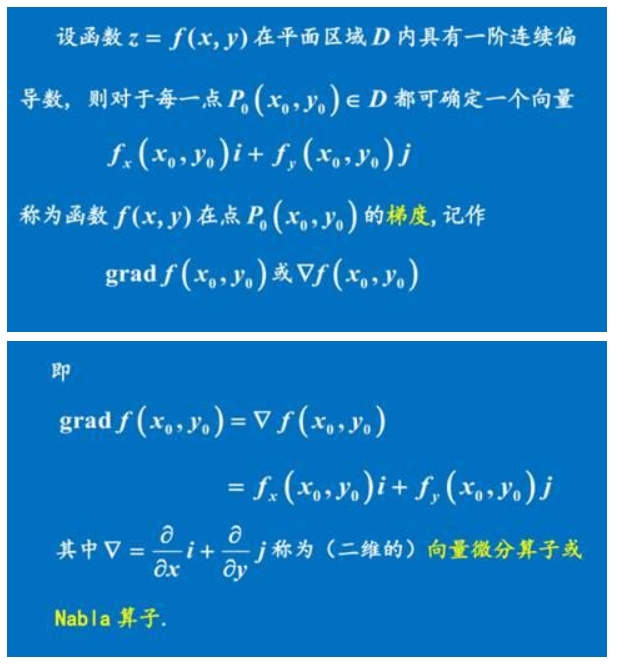看不懂也没关系，只要认识梯度的符号▽ 请看下面的推导过程：可以发现5.19和5.23很相似，就可以将5.19的推论应用到5.23来理解。 5.19：对x的变化更新，通过f(x)对x的导数（梯度）来描述。再乘以学习率。 5.23：对权值W的变化更新，通过ε对w的偏导（梯度）来描述。也是再乘以学习率。
展开全文深度学习 神经网络
• 但是接下来，我们会从梯度下降算法开始一步步计算到这个最小值！ 函数梯度为： 设置学习率： 设置初试起点为： 根据梯度下降法求下一个点的位置：  注， 时的函数值，小于 时的函数值。 ...
本篇博客来自其他博客以及论文消化吸收，假如读者熟悉BP网络正向传播，但是一直疑惑反向传播，那么可以继续看。
资料来源：
https://www.jianshu.com/p/c7e642877b0e
http://www.cnblogs.com/charlotte77/p/5629865.html
假设一个网络如下：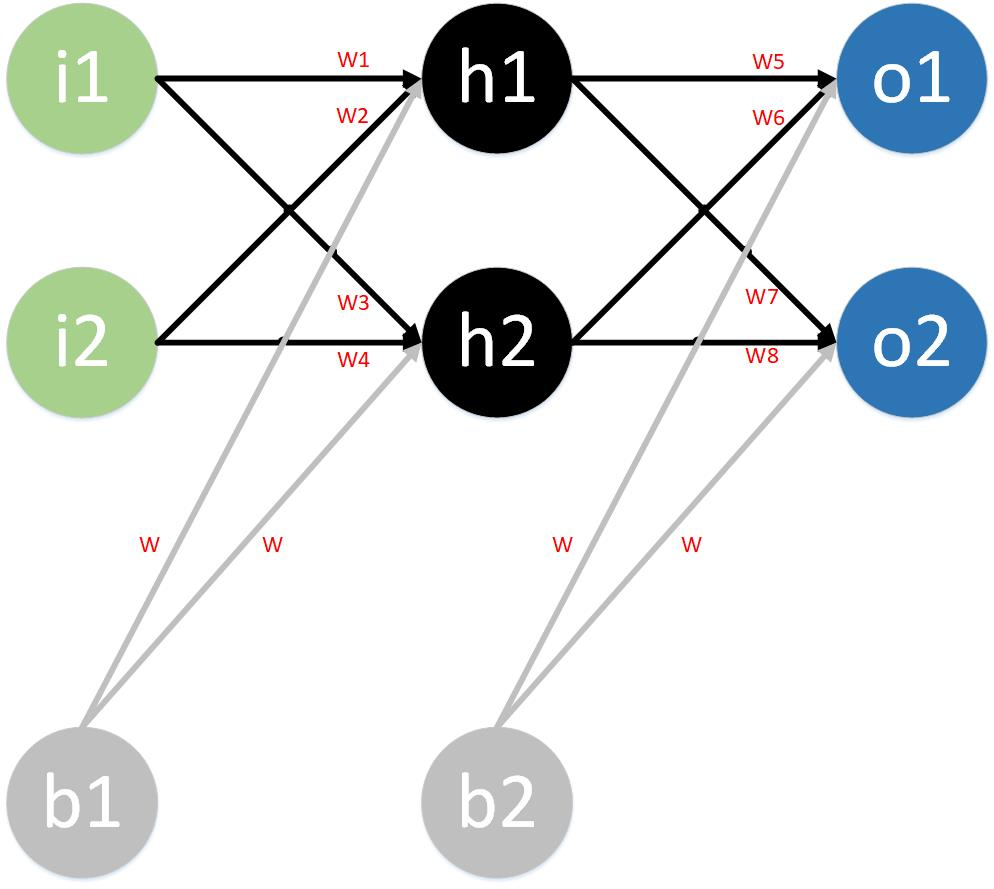第一层是输入层，包含两个神经元i1，i2，和截距项b1；注：截距项类似中的常数项c。
第二层是隐含层，包含两个神经元h1,h2和截距项b2；
第三层是输出层，包含两个神经元o1,o2。
每条线上标的wi是层与层之间连接的权重，激活函数我们默认为sigmoid函数。
现在对他们赋上初值，如下图：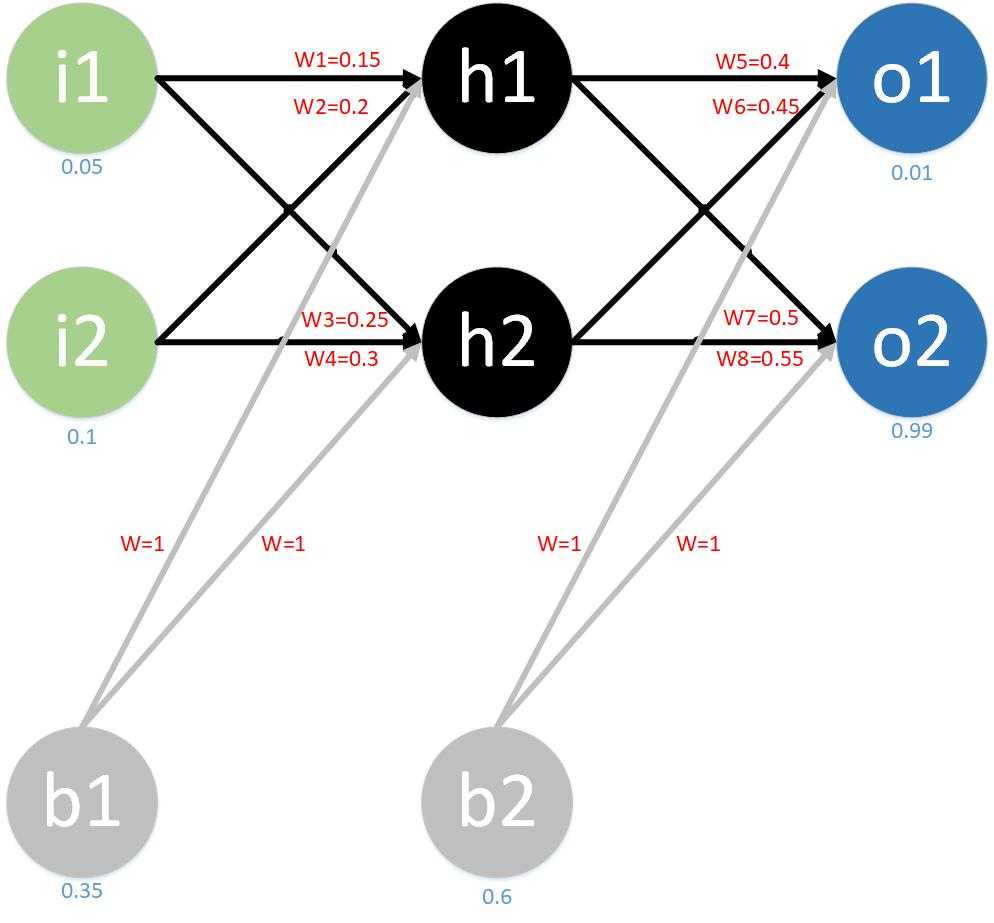其中，输入数据  i1=0.05，i2=0.1;
输出数据 o1=0.01,o2=0.99;
初始权重  w1=0.15,w2=0.2,w3=0.25,w4=0.3;
w5=0.4,w6=0.45,w7=0.5,w8=0.55;
w=1
目标：给出输入数据i1,i2(0.05和0.1)，使输出尽可能与原始输出o1,o2(0.01和0.99)接近。

Step 1 前向传播
1.输入层······>隐含层：
计算神经元h1的输入加权和：

计算神经元h1的输出:(此处用到激活函数为sigmoid函数)：

同理：
计算神经元h2的输入加权和：

计算神经元h2的输出:(此处用到激活函数为sigmoid函数)：

2.隐含层---->输出层：
计算神经元o1的输入加权和：

计算神经元o2的输出:(此处用到激活函数为sigmoid函数)：

计算神经元o2的输入加权和：

计算神经元o2的输出:(此处用到激活函数为sigmoid函数)：

这样前向传播的过程就结束了，我们得到输出值为[0.75136507 , 0.772928465]，与实际值[0.01 , 0.99]相差还很远，现在我们对误差进行反向传播，更新权值，重新计算输出。

Step 2 反向传播
1.计算总误差
总误差：(square error)，取误差平方和然后除以2，为的是方便后面求导。

但是有两个输出，所以分别计算o1和o2的误差，总误差为两者之和：

问题来了：怎么样才能使得取得最小值呢？？
解析：
(1)可以看成是嵌套的函数,嵌套关系如下（中间有省略，示意即可）：

(2）从上述关系可以发现，忽略中间关系，宏观上可以看做是i1，i2作为变量，而w1,w2,w3,w4,w5,w6,w7.w8作为参数的函数。
(3)当输入数据时，i1和i2就有了具体值i1=0.01，i2=0.99。虽然w1,w2,w3,w4,w5,w6,w7.w8此时有值，但是可以将其看作是变量，将看做是关于w1,w2,w3,w4,w5,w6,w7.w8的一个函数，问题就转换成了求当为最小值时w1,w2,w3,w4,w5,w6,w7.w8的取值。
(4)的最小值并不好找，所以借助高数中的梯度来一点点向的最小值靠近。
(4)举例，设函数，x,y为变量，m,n为参数，令x=2,y=6则函数变为,求取得最小值时m，和n的值。

补充：如何用梯度寻找最小值。
(1)梯度方向指的是函数值上升最快的方向，这个方向是局部的，什么意思？假设当前点沿着梯度方向走1米一直都是上升最快的，但是1米以后不再上升最快，梯度方向可能就变了，这也是为什么要设置学习率(步长)的原因。
(2)另外，由于我们要的是最小值，应该找梯度下降最快的方向。所以梯度方向要取反，朝着最快的方向趋向最小值。
(3)梯度下降法有将求解带入局部最小值的可能。
(4)梯度概念就是求偏导数，本例中就是分别对w1,w2,w3,w4,w5,w6,w7.w8求偏导数，这8个偏导数就是8个维度上的长度值，他们合向量方向就是梯度的方向。如果不理解，该补一补基础了，参考博文：https://blog.csdn.net/u010916338/article/details/81288309
(5)梯度下降算法单变量函数举例
假设单变量函数：

函数求导，可看做梯度：

设学习率为：

设初试点为：
注：0只是标记第一个位置，无其他含义。
根据梯度下降公式,下一个点的位置为：
注，时的函数值，小于时的函数值。
注，时的函数值，小于时的函数值。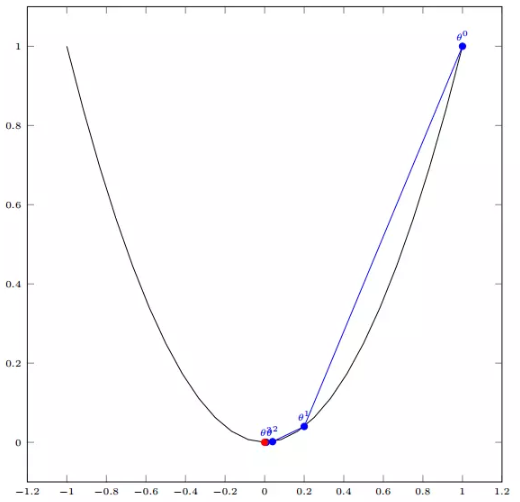(6)梯度下降算法多变量函数举例
假设多变量函数：

我们通过观察就能发现最小值其实就是 (0，0)点。但是接下来，我们会从梯度下降算法开始一步步计算到这个最小值！
函数梯度为：

设置学习率：

设置初试起点为：

根据梯度下降法求下一个点的位置：
注，时的函数值，小于时的函数值。
注，时的函数值，小于时的函数值。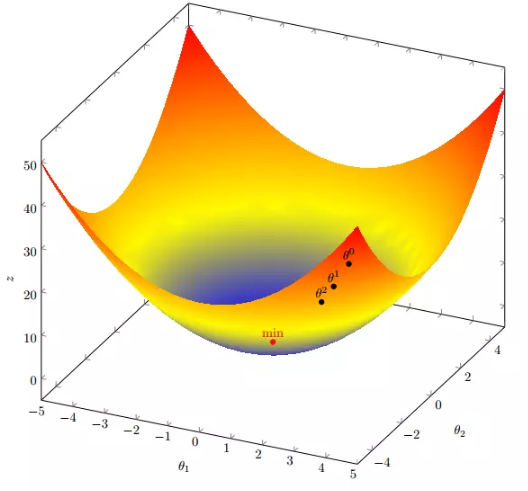2.隐含层······>输出层的权值更新：
以修改权重参数w5为例，先用整体误差对w5求偏导（链式法则），为什么这样求后续再介绍。

现在我们来分别计算每个式子的值：
计算：

计算：

补充：sigmod求导过程：

计算：

最后三者相乘：

更新w5权重值(是学习率，这里取值0.5)：

同理更新w6,w7,w8:

另外一点比较重要，如下所示：

令
则
用来表示输出层误差（显然输出层误差并不是这样的，如此表示是因为后续隐藏层的误差没法计算，是为了形式上的统一。具体用途结合后续隐藏层权重修改部分理解。）
注：简化公式的原因是为了编码的统一，为的是神经网络层统一定义，显然输出层的误差很好理解，但是隐藏层的误差不好理解，但是可以在形式上统一。

3.隐含层······>隐含层的权值更新：
以修改权重参数w1为例，先用整体误差对w1求偏导（链式法则），为什么这样求后续再介绍。

现在我们来分别计算每个式子的值：
计算：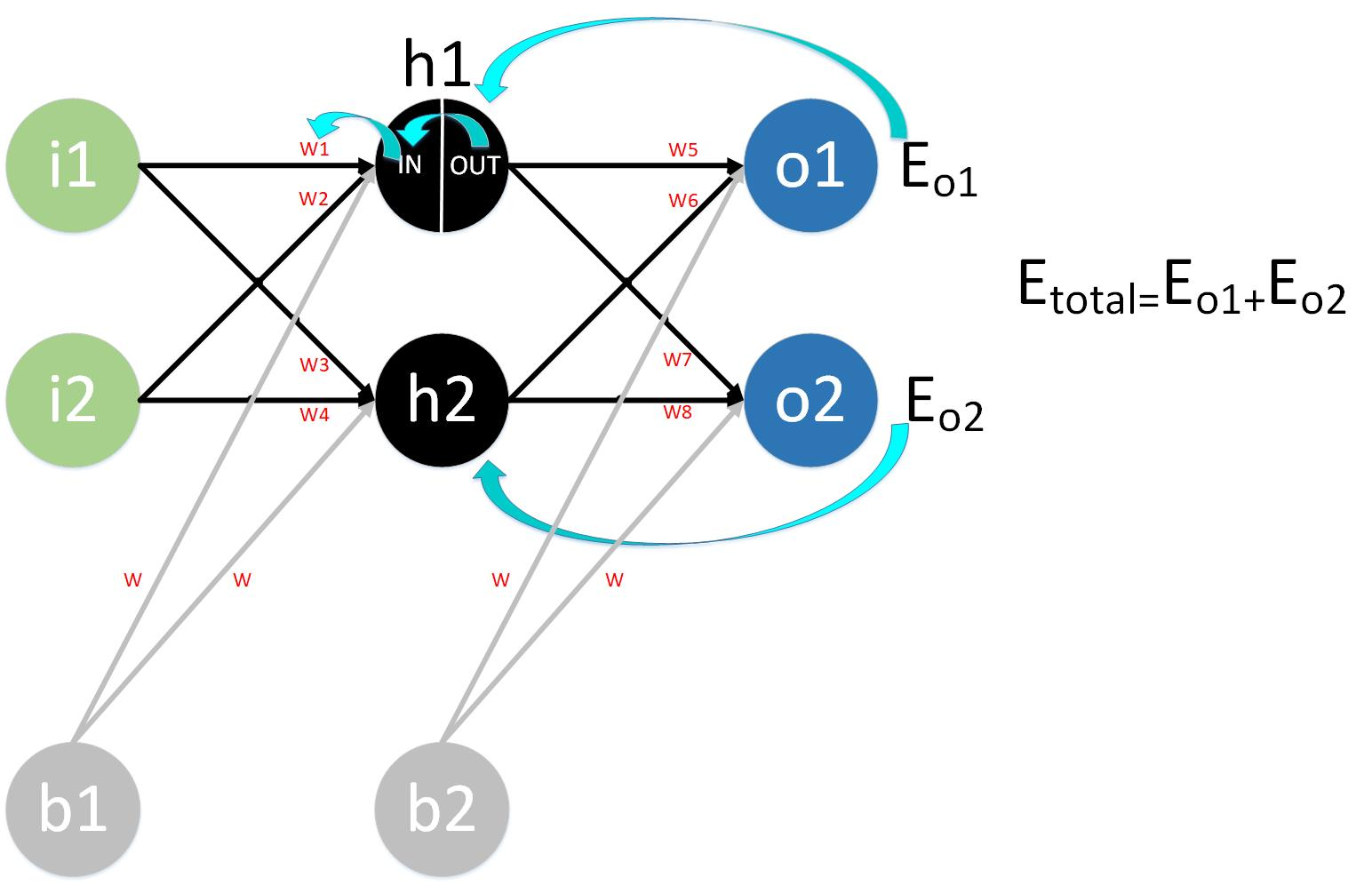因为到最后总误差计算有岔路，所以没有办法用链式求导法则进行统一的求导计算，两条路用的加权和函数不同，函数形式相同，但是参数不同。
解决思路如下，将整体误差拆分成两个小误差进行计算。

计算：

同理计算出：

则：

计算：

计算：

最后三者相乘：

更新w1权重值(是学习率，这里取值0.5)：

同理更新w2,w3,w4:

公式简化

（o为输出层神经元编号）
(解析：为隐藏层···>输出层的权重，因为求导，所以剩下的就只有权重，左半边的依然和输出层的公式简写一致，表示的是输出层的误差。)
(将看作是隐藏层的误差，记为：，注意只是为了形式保持一致，便于编码。)
注：结合输出层公式简写来看，输出层和隐藏层对于权重的偏导数都可以简写成：误差乘以上层传入的数据（误差形式不同分别定义即可，但对于权重的偏导数却可以统一定义。）

注意：
这样一次误差反向传播法就完成了，那么是不是可以接着输入新数据了呢？
不是这样的，刚才输入的数据i1=0.05，i2=0.1还要在进行一次正向传输，如果输出误差仍然在可接受范围之外，那么就会一直循环上述操作，再反向传播，再正向传播。直到把误差压缩在可接受范围之内时结束，为了防止迭代次数过多而一直循环，还要设置迭代次数，当迭代次数用尽之后，也要自动跳出迭代。
在这个例子中第一次迭代之后，总误差E(total)由0.298371109下降至0.291027924。迭代10000次后，总误差为0.000035085，输出为[0.015912196,0.984065734](原输入为[0.01,0.99]),证明效果还是不错的。
python代码实现：
# -*- coding: utf-8 -*-
"""
Created on Thu Oct 25 16:56:30 2018

@author: hrh
"""
#博客地址：http://www.cnblogs.com/charlotte77/p/5629865.html

#coding:utf-8
import random
import math

#
#   参数解释：
#   "pd_" ：偏导的前缀
#   "d_" ：导数的前缀
#   "w_ho" ：隐含层到输出层的权重系数索引
#   "w_ih" ：输入层到隐含层的权重系数的索引

#定义整个神经网络
class NeuralNetwork:

#设置步长，学习率
LEARNING_RATE = 0.5

def __init__(self, num_inputs, num_hidden, num_outputs, hidden_layer_weights = None, hidden_layer_bias = None, output_layer_weights = None, output_layer_bias = None):
self.num_inputs = num_inputs
#构造一个隐藏层
self.hidden_layer = NeuronLayer(num_hidden, hidden_layer_bias)
#构造一个输出层
self.output_layer = NeuronLayer(num_outputs, output_layer_bias)
#初始化输入层到隐藏层权重
self.init_weights_from_inputs_to_hidden_layer_neurons(hidden_layer_weights)
#初始化隐藏层到输出层权重
self.init_weights_from_hidden_layer_neurons_to_output_layer_neurons(output_layer_weights)

#初始化进入隐藏层的权重
def init_weights_from_inputs_to_hidden_layer_neurons(self, hidden_layer_weights):#hidden_layer_weights是个列表
weight_num = 0
for h in range(len(self.hidden_layer.neurons)):#隐藏层神经元个数
for i in range(self.num_inputs):#输入层神经元个数
if not hidden_layer_weights:
self.hidden_layer.neurons[h].weights.append(random.random())
else:
self.hidden_layer.neurons[h].weights.append(hidden_layer_weights[weight_num])#估计是有些权重需要提前设定
weight_num += 1

#初始化进入输出层的权重
def init_weights_from_hidden_layer_neurons_to_output_layer_neurons(self, output_layer_weights):
weight_num = 0
for o in range(len(self.output_layer.neurons)):
for h in range(len(self.hidden_layer.neurons)):
if not output_layer_weights:
self.output_layer.neurons[o].weights.append(random.random())
else:
self.output_layer.neurons[o].weights.append(output_layer_weights[weight_num])
weight_num += 1

def inspect(self):
print('------')
print('* Inputs: {}'.format(self.num_inputs))
print('------')
print('Hidden Layer')
self.hidden_layer.inspect()
print('------')
print('* Output Layer')
self.output_layer.inspect()
print('------')

#前向传播
def feed_forward(self, inputs):
hidden_layer_outputs = self.hidden_layer.feed_forward(inputs)
return self.output_layer.feed_forward(hidden_layer_outputs)

def train(self, training_inputs, training_outputs):
self.feed_forward(training_inputs)

#1. 求输出误差error对上一层神经元in的偏导数
pd_errors_wrt_output_neuron_total_net_input =  * len(self.output_layer.neurons)
for o in range(len(self.output_layer.neurons)):
#求输出误差error对上一层神经元in的偏导数
pd_errors_wrt_output_neuron_total_net_input[o] = self.output_layer.neurons[o].calculate_pd_error_wrt_total_net_input(training_outputs[o])

#2. 求隐藏层误差，求隐藏层误差error对于上层input的偏导数。
pd_errors_wrt_hidden_neuron_total_net_input =  * len(self.hidden_layer.neurons)
for h in range(len(self.hidden_layer.neurons)):
d_error_wrt_hidden_neuron_output = 0
for o in range(len(self.output_layer.neurons)):
#此处为计算隐藏层的输出误差，其实并不是真正的误差，只是在公式形式上和输出层误差形式类似。具体解释需要看博客。
d_error_wrt_hidden_neuron_output += pd_errors_wrt_output_neuron_total_net_input[o] * self.output_layer.neurons[o].weights[h]
#隐藏层总误差对于隐藏层输入input的偏导数。
pd_errors_wrt_hidden_neuron_total_net_input[h] = d_error_wrt_hidden_neuron_output * self.hidden_layer.neurons[h].calculate_pd_total_net_input_wrt_input()

#3. 修改隐藏层到输出层的权重。
for o in range(len(self.output_layer.neurons)):
for w_ho in range(len(self.output_layer.neurons[o].weights)):
#误差对输出层权重的偏导数。
pd_error_wrt_weight = pd_errors_wrt_output_neuron_total_net_input[o] * self.output_layer.neurons[o].calculate_pd_total_net_input_wrt_weight(w_ho)
# Δw = α * ∂Eⱼ/∂wᵢ根据学习率修改输出层神经元权重。
self.output_layer.neurons[o].weights[w_ho] -= self.LEARNING_RATE * pd_error_wrt_weight

#4. 更新隐含层的权重系数
for h in range(len(self.hidden_layer.neurons)):
for w_ih in range(len(self.hidden_layer.neurons[h].weights)):
#计算隐藏层误差对于输入层到隐藏层的权重的偏导数。
pd_error_wrt_weight = pd_errors_wrt_hidden_neuron_total_net_input[h] * self.hidden_layer.neurons[h].calculate_pd_total_net_input_wrt_weight(w_ih)
# Δw = α * ∂Eⱼ/∂wᵢ根据学习率修改隐藏层神经元权重。
self.hidden_layer.neurons[h].weights[w_ih] -= self.LEARNING_RATE * pd_error_wrt_weight

def calculate_total_error(self, training_sets):
total_error = 0
for t in range(len(training_sets)):
training_inputs, training_outputs = training_sets[t]#list中的元素还是一个list
self.feed_forward(training_inputs)
for o in range(len(training_outputs)):
total_error += self.output_layer.neurons[o].calculate_error(training_outputs[o])

#定义一层神经元
class NeuronLayer:

def __init__(self, num_neurons, bias):
#偏置值
self.bias = bias if bias else random.random()#python三目运算符
#定义神经元列表
self.neurons = []
#按照神经元个数初始化神经元列表，偏置值作为属性赋值给所有的神经元。
for i in range(num_neurons):
self.neurons.append(Neuron(self.bias))

def inspect(self):
print('Neurons:', len(self.neurons))
for n in range(len(self.neurons)):
print(' Neuron', n)
for w in range(len(self.neurons[n].weights)):
print('  Weight:', self.neurons[n].weights[w])
print('  Bias:', self.bias)

#一层神经元的前向输出
def feed_forward(self, inputs):
outputs = []
for neuron in self.neurons:
outputs.append(neuron.calculate_output(inputs))
return outputs

#一层神经元的前向输出，与上个函数功能相同，目前用途未知？？？？？？
def get_outputs(self):
outputs = []
for neuron in self.neurons:
outputs.append(neuron.output)
return outputs

#定义单个神经元
class Neuron:

#初始化设置截距
def __init__(self, bias):
#偏置
self.bias = bias
#上一层连接神经元的权重
self.weights = []

#计算神经元输出
def calculate_output(self, inputs):
#上一层输出都视为input，inputs是一个列表
self.inputs = inputs
#经过激活函数后的输出
self.output = self.squash(self.calculate_total_net_input())
return self.output

#计算神经元输入的加权平均和
def calculate_total_net_input(self):
total = 0
for i in range(len(self.inputs)):
#上一层都视为input，权重指的是上一层连接神经元的连接权重，而不是下一层连接神经元的权重。
total += self.inputs[i] * self.weights[i]
#最后每个神经元的总input都加上偏置项

# 定义激活函数
def squash(self, total_net_input):
return 1 / (1 + math.exp(-total_net_input))

#求输出误差error对上一层神经元in的偏导数
def calculate_pd_error_wrt_total_net_input(self, target_output):
return self.calculate_pd_error_wrt_output(target_output) * self.calculate_pd_total_net_input_wrt_input();

#计算神经元输出与目标值误差计算
def calculate_error(self, target_output):
return 0.5 * (target_output - self.output) ** 2

#求输出误差error对神经元out的偏导数
def calculate_pd_error_wrt_output(self, target_output):
return - (target_output - self.output)

#求神经元输出out对于输入in的偏导数
def calculate_pd_total_net_input_wrt_input(self):
return self.output * (1 - self.output)

#求输入in对权重w的偏导数，求完以后其实就是上层的输入值
def calculate_pd_total_net_input_wrt_weight(self, index):
return self.inputs[index]

# 文中的例子:

nn = NeuralNetwork(2, 2, 2, hidden_layer_weights=[0.15, 0.2, 0.25, 0.3], hidden_layer_bias=0.35, output_layer_weights=[0.4, 0.45, 0.5, 0.55], output_layer_bias=0.6)

for i in range(10000):
nn.train([0.05, 0.1], [0.01, 0.09])
print(i, round(nn.calculate_total_error([[[0.05, 0.1], [0.01, 0.09]]]), 9))




展开全文BP算法
•机器学习 python 人工智能
• 一直对ML中各种数学推导...1.梯度下降是ML中应用最广泛的用于求解模型参数的算法（类似算法还有牛顿法、拟牛顿法、共轭方向、共轭梯度法等）。原理主要是对损失函数的每一个函数求导，并利用负梯度对权值w进行更新。
• 梯度下降数学解释： 场景假设 梯度下降法的基本思想可以类比为...这个时候，便可利用梯度下降算法来帮助自己下山。怎么做呢，首先以他当前的所处的位置为基准，寻找这个位置最陡峭的地方，然后朝着下降方向走一步...
• 该视频是2014年拍的，虽然有点老，但理论却并不过时，是非常经典的机器学习入门教程，也正是因为这是入门教程，所以视频中的有些数学知识只给出了结论却未进行推导，这对于入门来说再适合不过了，但如果想深入学习...
• 梯度下降算法的正确步骤Title: What is the Gradient Descent Algorithm and its working. 标题:什么是梯度下降算法及其工作原理。 Gradient descent is a type of machine learning algorithm that helps us in ...深度学习 python 机器学习 人工智能
• 梯度下降法、随机梯度下降算法、批量梯度下降 梯度下降梯度下降就是我上面的推导，要留意，在梯度下降中，对于θ的更新，所有的样本都有贡献，也就是参与调整θ 其计算得到的是一个标准梯度。因而理论上来说一次...机器学习
• 传统的SVM使用凸二次规划的方式进行优化，使得损失函数收敛，参考李宏毅教授的机器学习课程的SVM的梯度下降的优化算法推导非常的简单明了，这里记录一下，并且参考Siraj Raval的例子使用梯度下降进行深入理解。...
• 梯度下降算法，本文是在学习涌井良幸先生的”深度学习的数学”一书后的笔记，仅用作个人学习和复习，由于笔者也是初学，所以难免会有各种错误，望各位大佬批评指正。 首先以二维函数举例： 对这个函数使用梯度下降...神经网络 机器学习 人工智能
• 用最简单的、通俗的语言教会小白学会“梯度下降算法的原理，并进行详细的公式推导，一步一步计算，代码一步一步讲解和操作，力求使小白“听得懂、学的会”人工智能 深度学习 语言线性回归机器学习 人工智能 深度学习
• 在深度网络的训练过程中，为了使损失尽可能的小，经常使用梯度下降算法训练网络，为什么沿着负梯度的方向更新权值就能使损失值越来越小了，下面给出梯度下降算法的简要推导。 已知函数f(x),当前x取值x0x0x_0,另x1...
• 在学习线性回归的时候很多课程都会讲到用梯度下降法求解参数，对于梯度下降算法怎么求出这个解讲的较少，自己实现一遍算法比较有助于理解算法，也能注意到比较细节的东西。具体的数学推导可以参照这一篇博客...python实现
• 自己学习吴恩达机器学习的总结，主要是关于梯度下降算法的一些推导与介绍，以及解释了，吴恩达课中没有解释推导的内容。
• 基于梯度下降算法的参数更新公式的推导 ​ 在前面一篇文章中写到了线性回归算法以及梯度下降算法对参数的更新： ​ θi=θi′−α∂Loss∂θ\theta_i=\theta_i^{&#x27;}-\alpha\frac{\partial Loss}{\...
• 人们在惊呼其效果之外，对其表现出如此效果的内在原理却知之甚少，为此，本文基于自己在之前课堂上学习到的知识对其内部工作情况做一个较为详细的介绍，考虑到目前主流机器学习还是基于随机梯度下降及BP算法进行网络...神经网络 机器学习
• Logistic回归cost函数的推导过程。...对于一个函数，我们要找它的最小值，有多种算法，这里我们选择比较容易用代码实现和符合机器学习步骤的梯度下降算法。 先来看看梯度下降算法中，自变量的迭代过程...
• 批量梯度下降、随机梯度下降、小批量梯度下降的python实现 之前写线性回归那一块内容的时候，发过手写二元线性回归梯度下降的博，由于基于方程的写法过于复杂，于是有了这一篇基于矩阵的梯度下降实现~python
• 小白学习课程：梯度下降算法与公式详细推导 国内“双一流”大学博士研究生，计算......